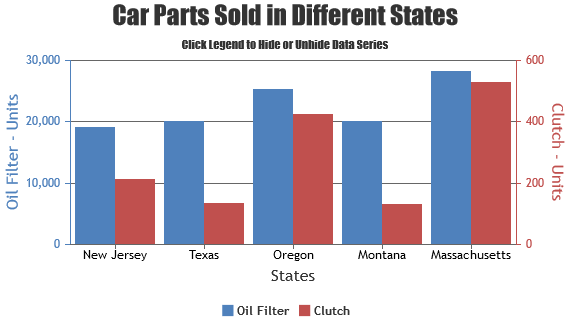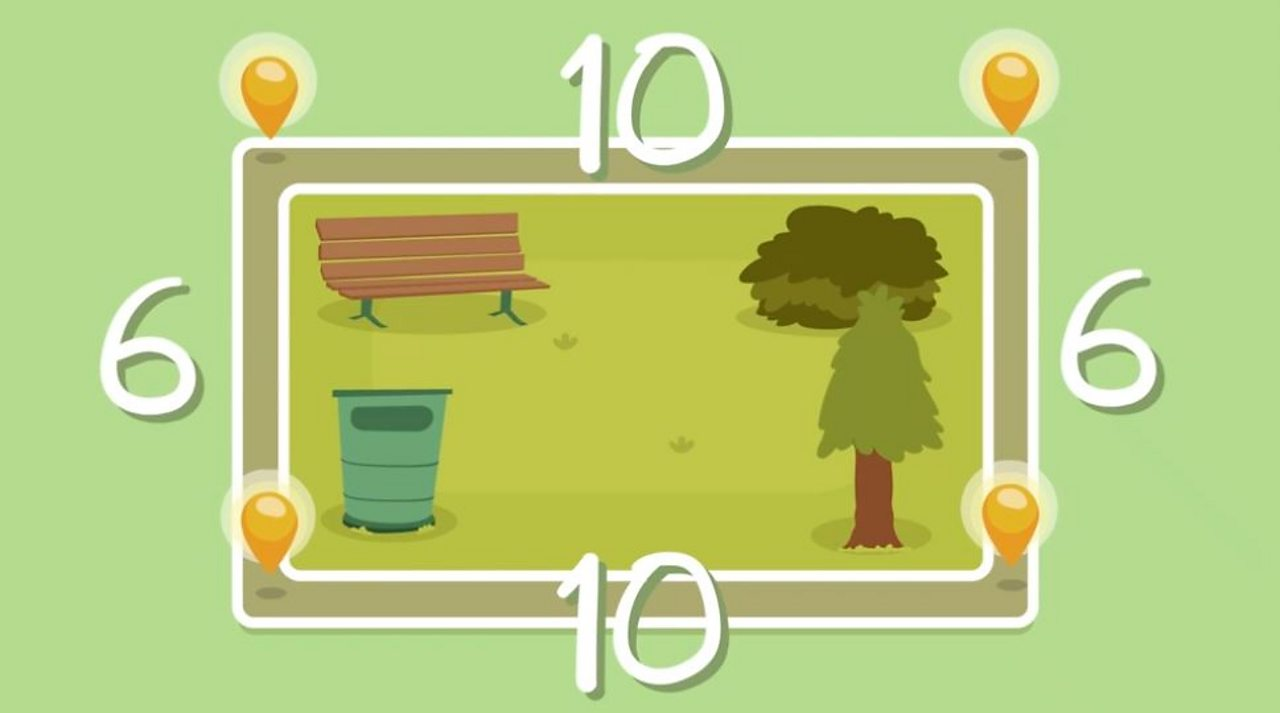## viernes, 30 de noviembre de 2018

### A Long Walk To Water

A Long Walk To WaterWatch the following videos in which Salva Dut, the protagonist, and Linda Sue Park, the author of the book, tells us his story... Which is not very different from the one written.

### Graphs using MS Excel

Graphs using MS ExcelClick on the following link to watch a tutorial on how to organise data in tables and graphs using MS Excel. If you already know how to do it, watch it to get more tips.

### Logos & Symbols

Logos & SymbolsWhat are logos and symbols? How are they used to communicate?
Here's a couple of clips which acn be used to answer those questions.

## viernes, 23 de noviembre de 2018

### Probability

ProbabilityThis is for you to practice probability for the upcoming evaluations.

Luciano M. 5A & Mr Luis

Probability Lesson
Game 1
Game 2

### Decimals

DecimalsWe have selected some of the best games on decimals so you can practice and learn more

## jueves, 18 de octubre de 2018

### Wonder

Wonder by J.R. Palacio

Any parts that you missed? Something you don't remember? Take a look at the following website. There are some summaries available for you to reinforce what you read.

## Area:

Calculating area:

To work out the area of a square or rectangle, multiply its height by its width.

If the height and width are in cm, the area is shown in cm². If the height and width are in m, the area is shown in m².

A square with sides of 5 m has an area of 25 m², because 5 × 5 = 25.

Visit the following links to practise and learn more:

Area 1

Area 2

Area 3

Area 4

## Perimetre:

Calculating perimeter:

The perimeter is the distance all the way around the outside of a 2D shape.

To work out the perimeter, add up the lengths of all the sidesThe perimeter of this shape is 10 + 10 + 6 + 6 = 32

Visit the following links to practise and learn more:

Perimeter 1

Perimeter 2

Perimeter 3

### Units of measurement (converting length units)

Basic conversion rules:

1) If you need to convert from a LARGER unit to a SMALLER unit, MULTIPLY.
For example: 5km into metres: 5 x 1000= 5.000 metres

2) If you need to convert from a SMALLER unit to a LARGER unit, DIVIDE.
For example: 10cm into metres: 10 / 100= 0,1 metres

## miércoles, 29 de agosto de 2018

### Lines, Polygons and Polyhedrons

Lines, Polygons and Polyhedrons

Visit the following links to practice and learn more.

Lines, segments, rays, parallel
Lines and intersections
Intersections
Identifying polygons
Matching Polygons
Interactive Quadrilaterals
Polyhedrons
Geometry facts